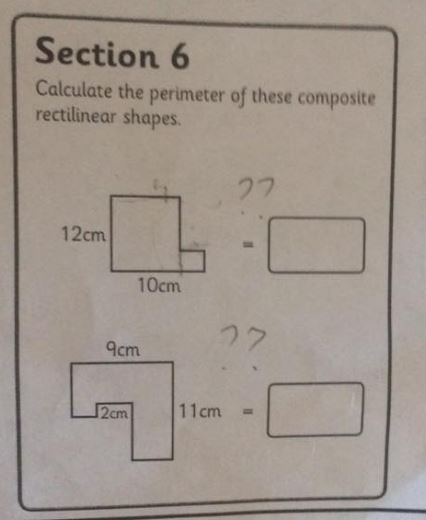# Can You Solve this Math Question for 10-year-olds?

•It seems as though the internet is going crazy over a tricky puzzle again, and this one isn't as easy as you may think. First posted by Manchester News on Twitter this geometry problem seems simple at first glance, but you soon realize that it actually involves some algebra. The problem was intended as a questi10-year-oldsar olds, but it is quickly stumping people much older. Take a look and see if you can figure it out.[Image Source: Manchester News]

There are two problems here, with the second being slightly harder than the first. One of the biggest debates that has raged through the comments section is what exactly the 2cm label is labeling. To clarify, the 2cm is labeling the side to its left. Now, with that clarification, can you work it out? The key to solving this problem is assigning equations of sides. Since we are solving for the perimeter, assign each varying side a number, and start working out some equations.

After working out each equation, you can subsitute in certain equations until there are no more unknowns and you can solve the problem. As a hint, the first problem is pure addition, and the second problem will require both addition and subtraction. Scroll down to the bottom of this post if you want to check your answers.

Most Popular

We know that many of our followers are pretty smart, but let us know in the comments if you could figure this one out without any help.

ANSWER: Both problems come out to a perimeter of 44SHOW COMMENT (1)